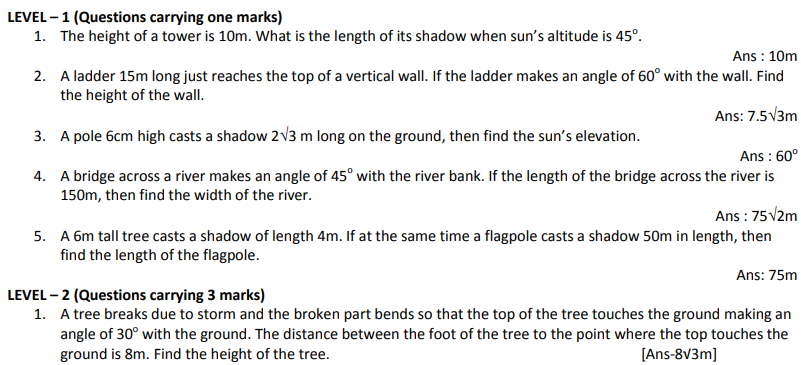# CBSE Class 10 Mathematics Trigonometry Assignment Set A

Read and download free pdf of CBSE Class 10 Mathematics Trigonometry Assignment Set A. Get printable school Assignments for Class 10 Mathematics. Standard 10 students should practise questions and answers given here for Chapter 9 Some Applications Of Trigonometry Mathematics in Grade 10 which will help them to strengthen their understanding of all important topics. Students should also download free pdf of Printable Worksheets for Class 10 Mathematics prepared as per the latest books and syllabus issued by NCERT, CBSE, KVS and do problems daily to score better marks in tests and examinations

## Assignment for Class 10 Mathematics Chapter 9 Some Applications Of Trigonometry

Class 10 Mathematics students should refer to the following printable assignment in Pdf for Chapter 9 Some Applications Of Trigonometry in standard 10. This test paper with questions and answers for Grade 10 Mathematics will be very useful for exams and help you to score good marks

### Chapter 9 Some Applications Of Trigonometry Class 10 Mathematics Assignment2. A kite is flying at a height of 60m above the ground. The string attached to the kite is temporarily tied to a point on the ground. The inclination of the string to the ground is 60o. Find the length of the string assuming that there is no slack in the string.
[Ans-40√3M]

The angle of elevation of the top of a hill at the foot of the tower is 60o and the angle of elevation of the top of  the tower from the foot of the hill is 30o. If the tower is 50m high, find the height of the hill. [Ans-150m]

LEVEL – 3

1. From the top of a 7m high building, the angle of elevation of the top of a cable tower is 60o and the angle of depression of the foot of the tower is 30o. Find the height of the tower. [Ans-28m]
2. The angle of elevation of an aeroplane from a point on the ground is 45o. After flight for 15 seconds the elevation changes to 30o. If the aeroplane is flying at a height of 3000m. Find the speed of the aeroplane.

[Ans-527.4km/h]

3. The angle of elevation of a cloud from a point h metres above a lake is α and the angle of derpression of its